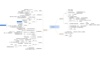pandas 订阅
pandas 是基于NumPy 的一种工具，该工具是为了解决数据分析任务而创建的。Pandas 纳入了大量库和一些标准的数据模型，提供了高效地操作大型数据集所需的工具。pandas提供了大量能使我们快速便捷地处理数据的函数和方法。你很快就会发现，它是使Python成为强大而高效的数据分析环境的重要因素之一。 展开全文pandas 是基于NumPy 的一种工具，该工具是为了解决数据分析任务而创建的。Pandas 纳入了大量库和一些标准的数据模型，提供了高效地操作大型数据集所需的工具。pandas提供了大量能使我们快速便捷地处理数据的函数和方法。你很快就会发现，它是使Python成为强大而高效的数据分析环境的重要因素之一。

pandas

PyData开发组

Python数据分析模块

AQR Capital Management
pandas简介
Pandas   是python的一个数据分析包，最初由AQR Capital Management于2008年4月开发，并于2009年底开源出来，目前由专注于Python数据包开发的PyData开发team继续开发和维护，属于PyData项目的一部分。Pandas最初被作为金融数据分析工具而开发出来，因此，pandas为时间序列分析提供了很好的支持。 Pandas的名称来自于面板数据（panel data）和python数据分析（data analysis）。panel data是经济学中关于多维数据集的一个术语，在Pandas中也提供了panel的数据类型。

• Pandas最好用的函数 Pandas是Python语言中非常好用的一种数据结构包， 包含了许多有用的数据操作方法。而且很多算法相关的库函数的输入数据结构都要求是pandas数据，或者有该数据的接口。 仔细看pandas的API...
Pandas最好用的函数
Pandas是Python语言中非常好用的一种数据结构包，包含了许多有用的数据操作方法。而且很多算法相关的库函数的输入数据结构都要求是pandas数据，或者有该数据的接口。
仔细看pandas的API说明文档，就会发现有好多有用的函数，比如非常常用的文件的读写函数就包括如下函数：
读取数据后，对于数据处理来说，有好多有用的相关操作的函数，但是我认为其中最好用的函数是下面这个函数：
apply函数
apply函数是pandas里面所有函数中自由度最高的函数。该函数如下：
DataFrame.apply(func, axis=0, broadcast=False, raw=False, reduce=None, args=(), **kwds)
该函数最有用的是第一个参数，这个参数是函数，相当于C/C++的函数指针。
这个函数需要自己实现，函数的传入参数根据axis来定，比如axis = 1，就会把一行数据作为Series的数据结构传入给自己实现的函数中，我们在函数中实现对Series不同属性之间的计算，返回一个结果，则apply函数会自动遍历每一行DataFrame的数据，最后将所有结果组合成一个Series数据结构并返回。
比如读取一个表格：假如我们想要得到表格中的PublishedTime和ReceivedTime属性之间的时间差数据，就可以使用下面的函数来实现：
import pandas as pd
import datetime   #用来计算日期差的包

def dataInterval(data1,data2):
d1 = datetime.datetime.strptime(data1, '%Y-%m-%d')
d2 = datetime.datetime.strptime(data2, '%Y-%m-%d')
delta = d1 - d2
return delta.days

def getInterval(arrLike):  #用来计算日期间隔天数的调用的函数
PublishedTime = arrLike['PublishedTime']
return days

if __name__ == '__main__':
fileName = "NS_new.xls";
df['TimeInterval'] = df.apply(getInterval , axis = 1)

有时候，我们想给自己实现的函数传递参数，就可以用的apply函数的*args和**kwds参数，比如同样的时间差函数，我希望自己传递时间差的标签，这样每次标签更改就不用修改自己实现的函数了，实现代码如下：
import pandas as pd
import datetime   #用来计算日期差的包

def dataInterval(data1,data2):
d1 = datetime.datetime.strptime(data1, '%Y-%m-%d')
d2 = datetime.datetime.strptime(data2, '%Y-%m-%d')
delta = d1 - d2
return delta.days

def getInterval_new(arrLike,before,after):  #用来计算日期间隔天数的调用的函数
before = arrLike[before]
after = arrLike[after]
days = dataInterval(after.strip(),before.strip())  #注意去掉两端空白
return days

if __name__ == '__main__':
fileName = "NS_new.xls";
df['TimeInterval'] = df.apply(getInterval_new ,
axis = 1, args = ('ReceivedTime','PublishedTime'))    #调用方式一
#下面的调用方式等价于上面的调用方式
df['TimeInterval'] = df.apply(getInterval_new ,
#下面的调用方式等价于上面的调用方式
df['TimeInterval'] = df.apply(getInterval_new ,

修改后的getInterval_new函数多了两个参数，这样我们在使用apply函数的时候要自己传递参数，代码中显示的三种传递方式都行。
最后，本篇的全部代码在下面这个网页可以下载：
https://github.com/Dongzhixiao/Python_Exercise/tree/master/pandas_apply
展开全文Python
• ## Pandas

千次阅读 2019-04-11 22:20:28
1:什么是pandas 定义：Pandas 纳入了大量库和一些标准的数据模型，提供了高效地操作大型数据集所需的工具。pandas提供了大量能使我们快速便捷地处理数据的函数和方法。 作用：numpy能够帮助我们处理数值，但是...
1:什么是pandas
定义：Pandas 纳入了大量库和一些标准的数据模型，提供了高效地操作大型数据集所需的工具。pandas提供了大量能使我们快速便捷地处理数据的函数和方法。
作用：numpy能够帮助我们处理数值，但是pandas除了处理数值之外（基于bumpy），还能够帮助我们处理其他类型数据。
2:pandas的常用数据类型
Series 一维，带标签数组              DataFrame 二维，Series容器

pd.Series([1,2,3,4], index=list('asdf'))

temp_dict = {'name': 'xiaohong', 'age': 18, 'tel':10086}
temp = pd.Series(temp_dict)
print(temp)
3:Series的切片和索引
Series对象本质上由两个数组构成，一个数组构成对象的键（index，索引），一个数组构成对象的值（values）， 键->值
ndarray的很多方法都可以运用于series类型，例如：argmax， clip，series也有where方法，但是结果跟ndarray不同。
t1 = pd.Series([1,2,3,4], index=list('asdf'))
#
temp_dict = temp_dict = {'name': 'xiaohong', 'age': 18, 'tel':10086}
t2 = pd.Series(temp_dict)
print(t2)

print(t1)
print(t1[0:])
print(t1[[1, 3]])
print(t2['age'])
print(t2[['age', 'tel']])
print(t2[[1, 2]])

# 当按照键取值没有的话会是NAN

print(t1.index)   # index类型可迭代  Index(['a', 's', 'd', 'f'], dtype='object') list(t1.index)
print(type(t1.index), len(t1.index))  # <class 'pandas.core.indexes.base.Index'> 4

print(t1.values)  # [1 2 3 4] object类型
print(type(t1.values))  # <class 'numpy.ndarray'>


展开全文• ## 使用pandas读取excel

万次阅读 多人点赞 2018-10-30 15:53:01
本文为作者原创，未经允许不得擅自转载。
Excel是微软的经典之作，在日常工作中的数据整理、分析和可视化方面，有其独到的优势，尤其在你熟练应用了函数和数据透视等高级功能之后，Excel可以大幅度提高你的工作效率。但如果数据量超大，Excel的劣势也就随之而来，甚至因为内存溢出无法打开文件，后续的分析更是难上加难。那么，有什么更好的解决办法吗？工欲善其事，必先利其器，在这里我们介绍使用Python的pandas数据分析包来解决此问题。
pd.read_excel(io, sheet_name=0, header=0, names=None, index_col=None,
usecols=None, squeeze=False,dtype=None, engine=None,
converters=None, true_values=None, false_values=None,
skiprows=None, nrows=None, na_values=None, parse_dates=False,
date_parser=None, thousands=None, comment=None, skipfooter=0,
convert_float=True, **kwds)


目录
1、io，Excel的存储路径
2、sheet_name，要读取的工作表名称
4、names， 自定义最终的列名
5、index_col， 用作索引的列
6、usecols，需要读取哪些列
7、squeeze，当数据仅包含一列
8、converters ，强制规定列数据类型
9、skiprows，跳过特定行
10、nrows ，需要读取的行数
11、skipfooter ， 跳过末尾n行

【文中使用英超、西甲的排名积分榜及射手榜作为原始数据~~~】

1、io，Excel的存储路径
建议使用英文路径以及英文命名方式。
import pandas as pd
io = r'C:\Users\Administrator\Desktop\data.xlsx'
2、sheet_name，要读取的工作表名称
可以是整型数字、列表名或SheetN，也可以是上述三种组成的列表。 整型数字：目标sheet所在的位置，以0为起始，比如sheet_name = 1代表第2个工作表。
data = pd.read_excel(io, sheet_name = 1)
data.head()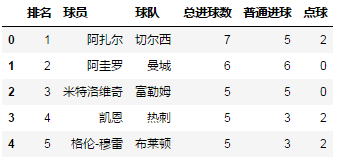列表名：目标sheet的名称，中英文皆可。
data = pd.read_excel(io, sheet_name = '英超射手榜')
data.head()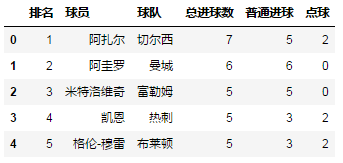SheetN：代表第N个sheet，S要大写，注意与整型数字的区别。
data = pd.read_excel(io, sheet_name = 'Sheet5')
data.head()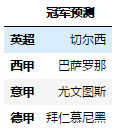组合列表： sheet_name = [0, '英超射手榜', 'Sheet4']，代表读取三个工作表，分别为第1个工作表、名为“英超射手榜”的工作表和第4个工作表。显然，Sheet4未经重命名。sheet_name 默认为0，取Excel第一个工作表。如果读取多个工作表，则显示表格的字典。对于初学者而言，建议每次读取一个工作表，然后进行二次整合。
data = pd.read_excel(io, sheet_name = ['英超积分榜', '西甲积分榜'], nrows = 5)
# sheet_name = ['英超积分榜', '西甲积分榜'] ，返回两个工作表组成的字典
data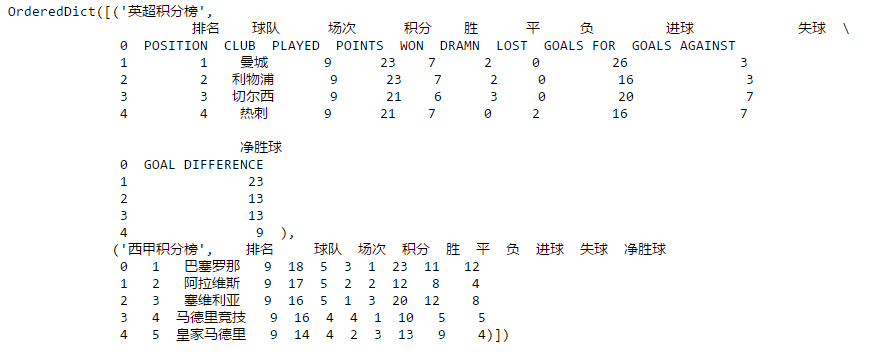默认为0 ，如果设置为[0,1]，则表示将前两行作为多重索引。
data = pd.read_excel(io, sheet_name = '英超积分榜', header = [0,1])
# 前两行作为列名。
data.head()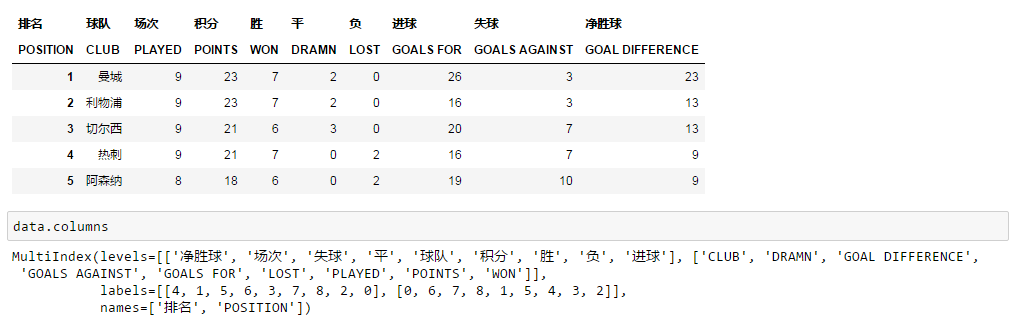4、names， 自定义最终的列名
一般适用于Excel缺少列名，或者需要重新定义列名的情况。注意：names的长度必须和Excel列长度一致，否则会报错。
data = pd.read_excel(io, sheet_name = '英超射手榜',
names = ['rank','player','club','goal','common_goal','penalty'])
data.head()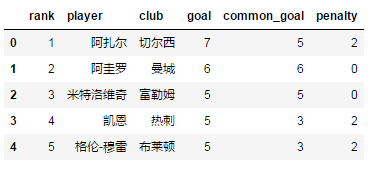5、index_col， 用作索引的列
可以是工作表列名称，如index_col = '排名'；可以是整型或整型列表，如index_col = 0 或 [0, 1]，如果选择多个列，则返回多重索引。
data = pd.read_excel(io, sheet_name = '英超射手榜', index_col = '排名')
data.head()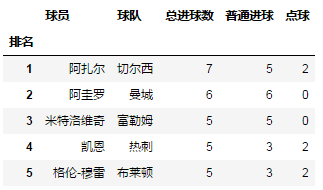data = pd.read_excel(io, sheet_name = '英超射手榜', index_col = [0, 1])
data.head()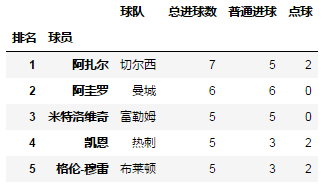6、usecols，需要读取哪些列
可以使用整型，从0开始，如[0,2,3]；可以使用Excel传统的列名“A”、“B”等字母，如“A：C, E” ="A, B, C, E"，注意两边都包括。usecols 可避免读取全量数据，而是以分析需求为导向选择特定数据，可以大幅提高效率。
data = pd.read_excel(io, sheet_name = '西甲射手榜', usecols = [0, 1, 3])
data.head()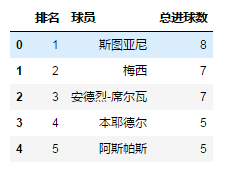data = pd.read_excel(io, sheet_name = '西甲射手榜', usecols = 'A:C, E')
# 啊？什么！！为啥不见C罗？？
# 大佬，C罗转会去尤文图斯啦~~~~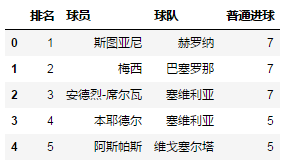7、squeeze，当数据仅包含一列
squeeze为True时，返回Series，反之返回DataFrame。
data = pd.read_excel(io, sheet_name = 'squeeze', squeeze  = True)
data.head()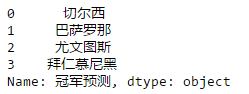data = pd.read_excel(io, sheet_name = 'squeeze', squeeze  = False)
data.head()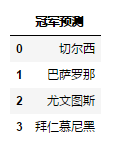8、converters ，强制规定列数据类型
converters = {'排名': str, '场次': int}， 将“排名”列数据类型强制规定为字符串（pandas默认将文本类的数据读取为整型），“场次”列强制规定为整型；  主要用途：保留以文本形式存储的数字。
data = pd.read_excel(io, sheet_name = 'converters')
data['排名'].dtype

data = pd.read_excel(io, sheet_name = 'converters', converters = {'排名': str, '场次': float})
data['排名'].dtype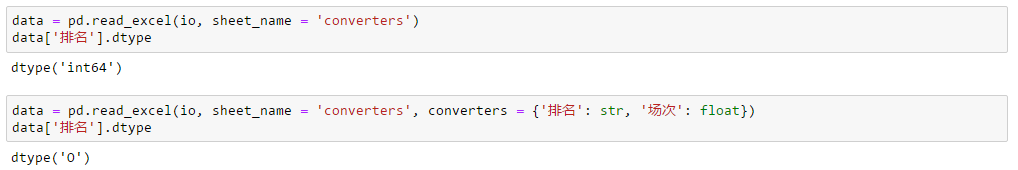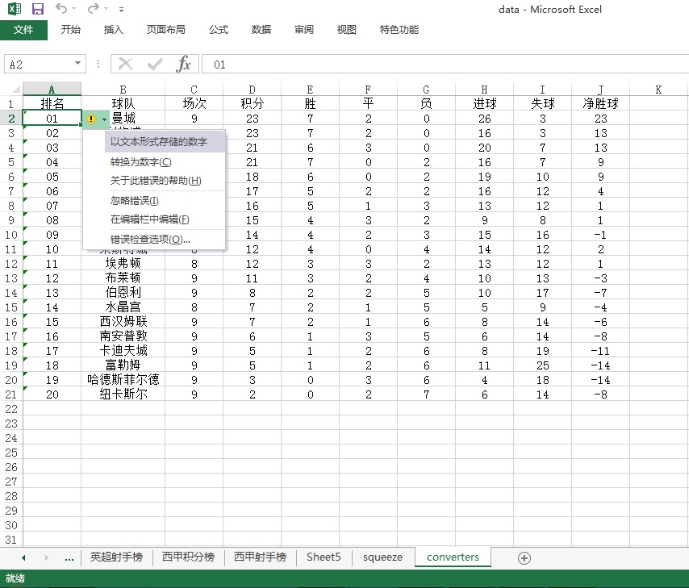9、skiprows，跳过特定行
skiprows= n， 跳过前n行； skiprows = [a, b, c]，跳过第a+1,b+1,c+1行（索引从0开始）；使用skiprows 后，有可能首行（即列名）也会被跳过。
data = pd.read_excel(io, sheet_name = '英超射手榜', skiprows = [1,2,3])
# 跳过第2,3,4行数据（索引从0开始，包括列名）
data.head()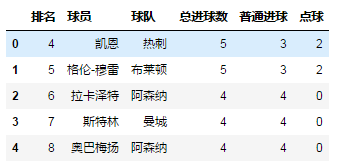data = pd.read_excel(io, sheet_name = '英超射手榜', skiprows = 3)
data.head()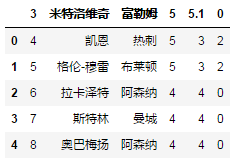10、nrows ，需要读取的行数
如果只想了解Excel的列名及概况，不必读取全量数据，nrows会十分有用。
data = pd.read_excel(io, sheet_name = '英超射手榜', nrows = 10)
data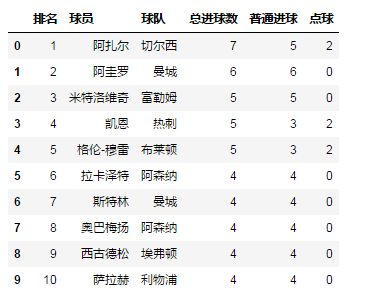11、skipfooter ， 跳过末尾n行
data = pd.read_excel(r'C:\Users\Administrator\Desktop\data.xlsx' ,
sheet_name = '英超射手榜', skipfooter = 43)
#  skipfooter = 43， 跳过末尾43行（索引从0开始）
data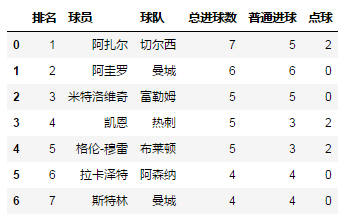欢迎搜索今日头条“海阔天空爱阅读”，欣赏更多文章~~
展开全文Python Excel
• ## Pandas DataFrame的基本属性详解

万次阅读 多人点赞 2019-06-24 19:13:33
Pandas DataFrame的一些基本属性 基本功能列表 import pandas as pd 导入库 df = pd.DataFrame(data=None, index=None, columns=None, dtype=None, copy=False) 创建一个DataFrame df.index df.columns df.axes df.T...
注：以下代码均在Jupyter中运行的。
基本功能列表
import pandas as pd 导入库
df = pd.DataFrame(data=None, index=None, columns=None, dtype=None, copy=False) 创建一个DataFrame
代码功能DataFrame()创建一个DataFrame对象df.values返回ndarray类型的对象df.iloc[ 行序,列序 ]按序值返回元素df.loc[ 行索引,列索引 ]按索引返回元素df.index获取行索引df.columns获取列索引df.axes获取行及列索引df.T行与列对调df. info()打印DataFrame对象的信息df.head(i)显示前 i 行数据df.tail(i)显示后 i 行数据df.describe()查看数据按列的统计信息
创建一个DataFrame
DataFrame()函数的参数index的值相当于行索引，若不手动赋值，将默认从0开始分配。columns的值相当于列索引，若不手动赋值，也将默认从0开始分配。
data = {
'性别':['男','女','女','男','男'],
'姓名':['小明','小红','小芳','大黑','张三'],
'年龄':[20,21,25,24,29]}
df = pd.DataFrame(data,index=['one','two','three','four','five'],
columns=['姓名','性别','年龄','职业'])
df

运行结果：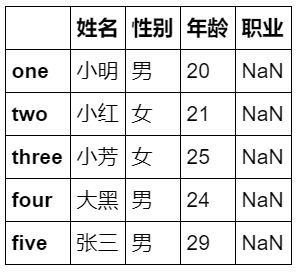df.values 返回ndarray类型的对象
ndarray类型即numpy的 N 维数组对象,通常将DataFrame类型的数据转换为ndarray类型的比较方便操作。如对DataFrame类型进行切片操作需要df.iloc[ : , 1:3]这种形式，对数组类型直接X[ : , 1:3]即可。
X = df.values
print(type(X)) #显示数据类型
X

运行结果：
<class 'numpy.ndarray'>
[['小明' '男' 20 nan]
['小红' '女' 21 nan]
['小芳' '女' 25 nan]
['大黑' '男' 24 nan]
['张三' '男' 29 nan]]

df.iloc[ 行序,列序 ] 按序值返回元素
df.iloc[1,1]

运行结果：
Index(['one', 'two', 'three', 'four', 'five'], dtype='object')

df.loc[ 行索引,列索引 ] 按索引返回元素
df.loc['one','性别']

运行结果：
男

df.index 获取行索引
df.index

运行结果：
Index(['one', 'two', 'three', 'four', 'five'], dtype='object')

df.columns 获取列索引
df.columns

运行结果：
Index(['姓名', '性别', '年龄', '职业'], dtype='object')

df.axes 获取行及列索引
df.axes

运行结果：
[Index(['one', 'two', 'three', 'four', 'five'], dtype='object'),
Index(['姓名', '性别', '年龄', '职业'], dtype='object')]

df.T index 与 columns 对调
df.T

运行结果：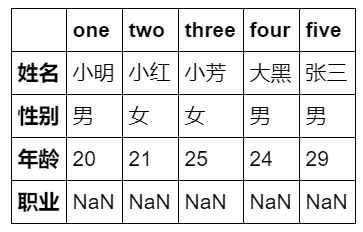df.info() 打印DataFrame对象的信息
df.info()

运行结果：
<class 'pandas.core.frame.DataFrame'>
Index: 5 entries, one to five
Data columns (total 4 columns):
姓名    5 non-null object
性别    5 non-null object
年龄    5 non-null int64
职业    0 non-null object
dtypes: int64(1), object(3)
memory usage: 200.0+ bytes


df.head(2)

运行结果：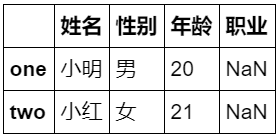若想要显示前几列数据，可用df.T.head(i)
df.tail(i) 显示后 i 行数据
df.tail(2)

运行结果：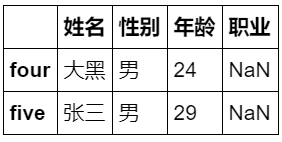df.describe() 查看数据按列的统计信息
可显示数据的数量、缺失值、最小最大数、平均值、分位数等信息
             年龄
count   5.000000
mean   23.800000
std     3.563706
min    20.000000
25%    21.000000
50%    24.000000
75%    25.000000
max    29.000000

展开全文Python
• 原因是最近xlrd更新到了2.0.1版本，只支持.xls文件。所以pandas.read_excel(‘xxx.xlsx’)会报错。 可以安装旧版xlrd，在cmd中运行： pip uninstall xlrd pip install xlrd==1.2.0python
• ## pandas apply() 函数用法

万次阅读 多人点赞 2019-08-22 10:00:46
理解 pandas 的函数，要对函数式编程有一定的概念和理解。函数式编程，包括函数式编程思维，当然是一个很复杂的话题，但对今天介绍的 apply() 函数，只需要理解：函数作为一个对象，能作为参数传递给其它函数，并且...
• pandas的数据 结构：Series、DataFrame、索引对象 pandas基本功能：重新索引，丢弃指定轴上的项，索引、选取和过滤，算术运算和数据对齐，函数应用和映射，排序和排名，带有重复值的轴索引 Pandas介绍 pandas含有...series dataframe
• ## pandas 删除指定行

万次阅读 2018-03-03 00:18:13
在处理pandas的DataFrame中，如果想像excel那样筛选，只要其中的某一行或者几行，可以使用isin()方法来实现，只需要将需要的行值以列表方式传入即可，还可传入字典，进行指定筛选。但是如果我们只想要所有内容中不...DataFrame
• ## pandas小记：pandas索引和选择

万次阅读 多人点赞 2014-01-08 21:49:08
检索/选择 索引选择时建议全部使用loc（尤其是修改df原本数据时），原因是最下面说的视图和显示拷贝。 dataframe列选择 和Series一样，在DataFrame中的一列可以通过字典记法或属性来检索，返回Series： ...python
• ## pandas DataFrame数据转为list

万次阅读 多人点赞 2017-05-21 22:46:10
首先使用np.array()函数把DataFrame转化为np.ndarray()，再利用tolist()函数把np.ndarray()转为list，示例代码如下：# -*- coding:...import pandas as pddata_x = pd.read_csv("E:/Tianchi/result/features.csv",usecocsv numpy 数据
• 输出格式控制 pandas dataframe数据全部输出，数据太多也不用省略号表示。 pd.set_option('display.max_columns',None) 或者 with option_context('display.max_rows', 10, 'dis...csv
• Get list from pandas DataFrame column headers - Pandas 获取列名 https://stackoverflow.com/questions/19482970/get-list-from-pandas-dataframe-column-headers 获取DataFrame的列名是一个比较简单的操作，又...python
• ## pandas的dataframe如何更改数据类型？

万次阅读 多人点赞 2018-07-22 20:38:16
pandas的dataframe数据类型转换 在使用pandas库进行数据分析时，有时候会需要将object类型转换成数值类型(float,int)，那么如何做呢？ 主要有以下三种方法：创建时指定类型，df.astype强制类型转换，以及使用pd....
• ## Pandas入门详细教程

万次阅读 多人点赞 2020-06-25 10:00:00
作者：luanhz来源：小数志导读本文主要是对pandas进行入门详细介绍，通过本文你将系统性了解pandas为何会有数据分析界"瑞士军刀"的盛誉。行文二级目录01 关于...
• pandas转数组 np.array(pandas) 数组转pandas pandas.DataFrame(numpy) pandas连接，只是左右接上，不合并值 df = pd.concat([suojindf,df], axis=1) 以上这篇连接pandas以及数组转pandas的方法就是小编分享给...
• import pandas as pd import numpy as np a=np.array([[1,2,3],[4,5,6],[7,8,9]]) df1=pd.DataFrame(a,index=['row0','row1','row2'],columns=list('ABC')) print(df1) df2=df1.copy() #删除/选取...python
• ## Pandas-排序函数sort_values()

万次阅读 多人点赞 2018-10-18 22:21:33
pandas中的sort_values()函数类似于SQL中order by的原理，可以将数据集依照某个字段中的数据进行排序。 二、sort_values()函数的具体参数 用法： DataFrame.sort_values(by=‘##’,axis=0,ascending=True, in...Python 排序
• ## python之pandas简单介绍及使用（一）

万次阅读 多人点赞 2019-06-13 11:00:45
一、　Pandas简介 1、Python Data Analysis Library 或 pandas 是基于NumPy 的一种工具，该工具是为了解决数据分析任务而创建的。Pandas 纳入了大量库和一些标准的数据模型，提供了高效地操作大型数据集所需的工具...python
• Pandas是python中非常常用的数据分析库，在数据分析，机器学习，深度学习等领域经常被使用。本课程会讲解到pandas中最核心的一些知识点，包括Series以及DataFrame的构建，赋值，操作，选择数据，合并等等，以及使用...python工程师 python爬虫 python python教程 python安装
• 面板数据{pandas数据结构有一维Series，二维DataFrame，这是三维Panel}pandas有一个Panel数据结构，可以将其看做一个三维版的，可以用一个由DataFrame对象组成的字典或一个三维ndarray来创建可视化
• ## [Python3]pandas.merge用法详解

万次阅读 多人点赞 2019-06-06 08:42:18
pandas提供了一组高级的、灵活的、高效的核心函数，能够轻松的将数据规整化。这节主要对pandas合并数据集的merge函数进行详解。(用过SQL或其他关系型数据库的可能会对这个方法比较熟悉。) 1.merge函数的参数一览表 ...Python merge 合并数据集 pd.merge
• apply 是 pandas 库的一个很重要的函数，多和 groupby 函数一起用，也可以直接用于 DataFrame 和 Series 对象。主要用于数据聚合运算，可以很方便的对分组进行现有的运算和自定义的运算。 数据集 使用的数据...python 数据分析
• Python、Anaconda、Pandas以及PyCharm的安装 文章来源：企鹅号 - Michael的笔记本 开发环境的搭建是一件入门比较头疼的事情，在上期的文稿基础上，增加一项Anaconda的安装介绍。Anaconda是Python的一个发行版本，...pycharm
• pandaspandaspandaspandaspandaspandaspandas（英文书）
• python安装pandas python安装pandas python安装pandas python安装pandas
• PANDAS 思维导图，PANDAS 思维导图，PANDAS 思维导图，PANDAS 思维导图，
• 在使用pandas的时候，有些场景需要对数据内部进行分组处理，如一组全校学生成绩的数据，我们想通过班级进行分组，或者再对班级分组后的性别进行分组来进行分析，这时通过pandas下的groupby()函数就可以解决。...
• ## pandas中根据列的值选取多行数据

万次阅读 多人点赞 2018-05-16 09:29:57
在excel或Mysql中经常会用到多列条件查询或筛选，下面一起来看看pandas怎么实现这一功能。
• pandas 中文手册,便于初学者大致了解pandas大致功能。官方教程是官方文档的教程页面上的教程。包括：十分钟搞定 pandasPandas 秘籍、学习 Pandas...